# Settling Time: What is it? (Formula And How To Find it in MATLAB)

Contents

## What is Settling Time?

The settling time of a dynamic system is defined as the time required for the output to reach and steady within a given tolerance band. It is denoted as Ts. Settling time comprises propagation delay and time required to reach the region of its final value. It includes the time to recover the overload condition incorporated with slew and steady near to the tolerance band.

The tolerance band is a maximum allowable range in which the output can be settle. Generally, the tolerance bands are 2% or 5%.

Settling time in step response of a second-order system is as shown in the below figure.

## Settling Time Formula

Settling time depends on natural frequency and response of the system. General equation of settling time is;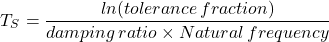The unit step response of second order system is expressed as;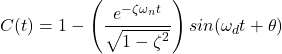This equation divides into two parts;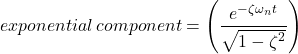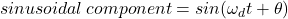To calculate the settling time, we only need the exponential component as it cancels the oscillatory part of sinusoidal component. And the tolerance fraction is equal to the exponential component.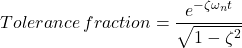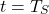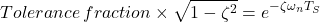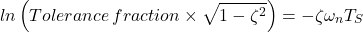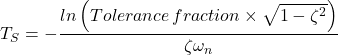## How to Calculate Settling Time

To calculate settling time, we consider a first order system with unit step response.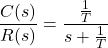For unit step response,Hence,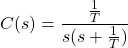Now, calculate the value for A1 and A2.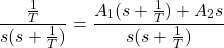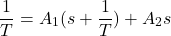Assume s = 0;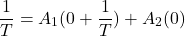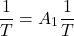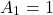Assume s = -1/T;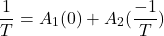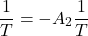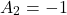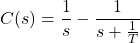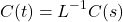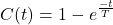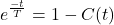For 2% error, 1-C(t) = 0.02;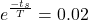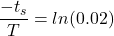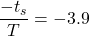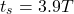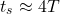This equation gives settling time for first order system with unit step input.

For second order system, we need to consider below equation;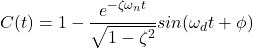In this equation, exponential term is important to find the value of settling time.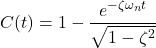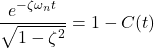Now, we consider 2% error. Therefore, 1 – C(t) = 0.02;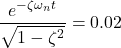The value of damping ratio (ξ) depends on the type of second order system. Here, we consider an underdamped second order system. And the value of ξ lies between 0 and 1.

So, denominator of above equation is nearly equal to 1. And to make an easy calculation, we can neglect it.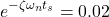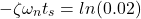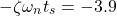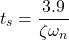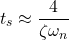This equation can be used only for 2% error band and underdamped second order system.

Similarly, for 5% error band; 1 – C(t) = 0.05;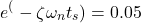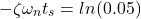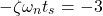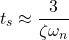For second order system, before finding settling time, we need to calculate the damping ratio.

## Root Locus Settling Time

Settling time can be calculated by the root locus method. Settling time depends on the damping ratio and natural frequency.

These quantities can be derived with the help of root locus method. And we can find the settling time.

Let’s understand with an example.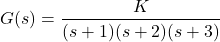And Overshoot = 20%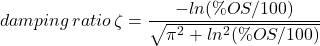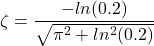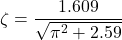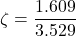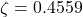From the root locus plot; you can find the dominant poles;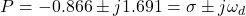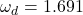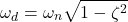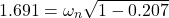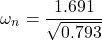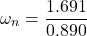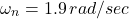Now, we have the value of ξ and ωn,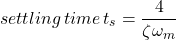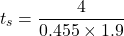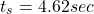The root locus plot is derived from MATLAB. For that use “sisotool”. Here, you can add a constraint for the percentage overshoot is equal to 20%. And get dominant poles easily.

The below figure shows the root locus plot from MATLAB.

We can find the settling time with the help of MATLAB. The unit step response of this system is as shown below figure.

## How to Reduce Settling Time

The settling time is the time required to achieve target. And for any control system, the settling time must be kept minimum.

Reduce the settling time is not an easy task. We need to design a controller to reduce the settling time.

As we know, there are three controllers; proportional (P), Integral (I), derivative (D). With a combination of these controllers, we can achieve our requirements of the system.

The gain of the controllers (KP, KI, KD) is chosen according to the system requirement.

Increase proportional gain KP, results in a small change in settling time. Increase in integral gain KI, settling time increases. And increase in derivative gain KD, settling time decreases.

Therefore, the derivative gain increases to decrease the setting time. While selecting the gain values of the PID controller, it may affect the other quantities also like rise time, overshoot, and Steady-state error.

## How to Find Settling Time in MATLAB

In MATLAB, settling time can be found by a step function. Let’s understand by example.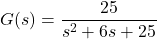First, we calculate the settling time by equation. For that, compare this transfer function with general transfer function of second order system.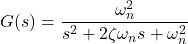Therefore,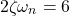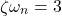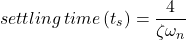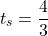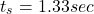This value is an approximate value as we have taken assumptions while calculating the equation of settling time. But in MATLAB, we get the exact value of settling time. So, this value may be slightly different in both cases.

Now, to calculate settling time in MATLAB, we use the step function.

clc; clear all; close all;

num = [0 0 25];
den = [1 6 25];

t = 0:0.005:5;

sys = tf(num,den);
F = step(sys,t);
H = stepinfo(F,t)

step(sys,t);

Output:

H =

RiseTime: 0.3708
SettlingTime: 1.1886
SettlingMin: 0.9071
SettlingMax: 1.0948
Overshoot: 9.4780
Undershoot: 0
Peak: 1.0948
PeakTime: 0.7850


And you get a graph of response as shown in the below figure.

In MATLAB, by default percentage band of error is 2%. You can change this in graph for different error band. for that, right-click on graph > properties > options > “show settling time within ___ %”.

Another way to find the settling time by running a loop. As we know, for the 2% error band, we consider the response between 0.98 to 1.02.

clc; clear all; close all;

num = [0 0 25];
den = [1 6 25];

t = 0:0.005:5;

[y,x,t] = step(num,den,t);

S = 1001;
while y(S)>0.98 & y(S)<1.02;
S=S-1;
end
settling_time = (S-1)*0.005

Output:

settling_time = 1.1886

Want To Learn Faster? 🎓
Get electrical articles delivered to your inbox every week.
No credit card required—it’s 100% free.